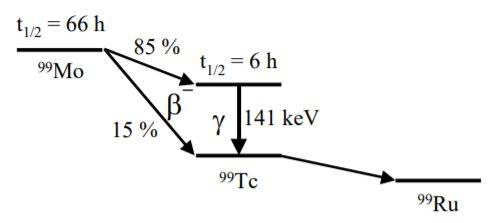# Nuclear decay scheme

Benlaww
Homework Statement:
Using a simplified decay scheme for the generator (shown in attempt of solution box) and assuming that initially only 99Mo is present, the number of 99Tc atoms N_Tc as a
function of time t is given by:
N_Tc(t) = (landa_Mo/(landa_Tc - landa_Mo))*N_Mo(0)*(e^(-landa_Mo*t) - e^(-landa_Tc*t))

Calculate the minimum activity of 99Mo required to produce 20 MBq of 99Tc at t
= 6 hours. State clearly any assumptions you make
Relevant Equations:
A=landa*NI'm not too sure where to start, do I differentiate the equation?

willem2
I'm not too sure where to start, do I differentiate the equation?
You can just calculate how much Tc99m has an acitivity of 30 Bq, and then use the equation to calculate how much Mo you need six hours earlier. The only thing not accounted for in the equation is the yield of 85% Tc99m.
I'm sure you can ignore the decay of the ordinary Tc99,

•Benlaww and Steve4Physics
Homework Helper
Gold Member
2022 Award
There's a mistake/ambiguity in the question which could cause confusion. There are 2 different forms of technetium referred to in this question but the question doesn't distinguish between them.

99Mo decays either to 99mTc (the metastable form) or to 99Tc.

Neither the diagram nor the question mention 99mTc but it appears you are actually being asked how many atoms of 99Mo were needed to produce 20MBq of 99mTc (not 20MBq of 99Tc).

I agree with @willem2's approach, except that I presume
"how much Tc99m has an acitivity of 30 Bq"
should really be
"how many atoms of Tc99m have an acitivity of 20MBq ".

Also, for information, the English-equivalent spelling of the Greek lettter ##\lambda## is ‘lambda’. It is pronounced ‘lam-da’. (Not to be confused with the dance the 'lambada' as some of my students did many years ago!).

•Benlaww